# Hashing Passwords in Python with BCrypt

• Last Updated : 03 Jun, 2022

In this article, we will see how to hash passwords in Python with BCrypt. Storing passwords in plain text is a bad practice as it is vulnerable to various hacking attempts. That’s why it is recommended to keep them in a hashed form.

## What is hashing?

It’s a process of converting one string to another using a hash function. There are various types of hash functions but there are some basic similarities that are satisfied by all of them is that hashing is an irreversible process. i.e. conversion should be only one way, the length of hash should be fixed, and an input string should uniquely correspond with a hash so that we can compare them later, this makes it ideal for passwords and authentication.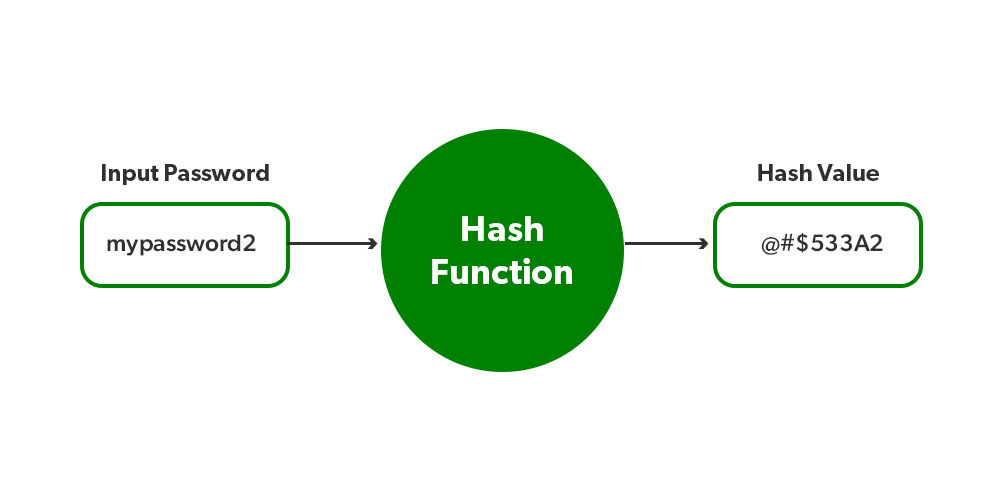## Hash a Password in Python Using Bcrypt

Bcrypt is a password hashing function designed by Nelis Provos and David Mazières. Bcrypt uses strong cryptography to hash and salts password based on the Blowfish cipher. To make encryption stronger we can increase the “cost factor” so it can be increased as computers become faster. It is also intended to be slow, to make the brute force attacks slower and harder.

To install Bcrypt use the command –

`pip install bcrypt`

The functions in Bcrypt used –

• bcrypt.gensalt() –  It is used to generate salt. Salt is a pseudorandom string that is added to the password. Since hashing always gives the same output for the same input so if someone has access to the database, hashing can be defeated. for that salt is added at end of the password before hashing. It doesn’t need any arguments and returns a pseudorandom string.
• bcrypt.hashpw() – It is used to create the final hash which is stored in a database.
• Arguments – We can pass Salt and Password in form of bytecode.
• Return value – If hashing is successful, it returns a hash string.

To use bcrypt, you’ll need to import bcrypt module, After that the bcrypt.hashpw() function takes 2 arguments: A string (bytes) and Salt. Salt is random data used in the hashing function. Let’s hash a password and print it in the following examples.

Example 1:

## Python3

 `import` `bcrypt ` ` `  `# example password ` `password ``=` `'password123'` ` `  `# converting password to array of bytes ` `bytes ``=` `password.encode(``'utf-8'``) ` ` `  `# generating the salt ` `salt ``=` `bcrypt.gensalt() ` ` `  `# Hashing the password ` `hash` `=` `bcrypt.hashpw(bytes, salt) ` ` `  `print``(``hash``)`

Output: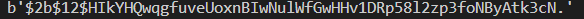Example 2:

Now let’s just change the input password a little bit to see the behavior of hashing.

## Python3

 `import` `bcrypt ` ` `  `# example password ` `password ``=` `'passwordabc'` ` `  `# converting password to array of bytes ` `bytes ``=` `password.encode(``'utf-8'``) ` ` `  `# generating the salt ` `salt ``=` `bcrypt.gensalt() ` ` `  `# Hashing the password ` `hash` `=` `bcrypt.hashpw(bytes, salt) ` ` `  `print``(``hash``)`

Output: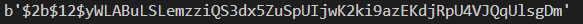The following example checks a password against a hashed value.

Example 1:

Here we will check whether the user has entered the correct password or not, for that we can use bcrypt.checkpw(password, hash). At first, let’s assume the user entered the wrong password.

## Python3

 `import` `bcrypt ` ` `  `# example password ` `password ``=` `'passwordabc'` ` `  `# converting password to array of bytes ` `bytes ``=` `password.encode(``'utf-8'``) ` ` `  `# generating the salt ` `salt ``=` `bcrypt.gensalt() ` ` `  `# Hashing the password ` `hash` `=` `bcrypt.hashpw(bytes, salt) ` ` `  `# Taking user entered password  ` `userPassword ``=`  `'password000'` ` `  `# encoding user password ` `userBytes ``=` `userPassword.encode(``'utf-8'``) ` ` `  `# checking password ` `result ``=` `bcrypt.checkpw(userBytes, ``hash``) ` ` `  `print``(result)`

Output: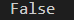Example 2:

Now let’s see what happens when passwords are matched:

## Python3

 `import` `bcrypt ` ` `  `# example password ` `password ``=` `'passwordabc'` ` `  `# converting password to array of bytes ` `bytes ``=` `password.encode(``'utf-8'``) ` ` `  `# generating the salt ` `salt ``=` `bcrypt.gensalt() ` ` `  `# Hashing the password ` `hash` `=` `bcrypt.hashpw(bytes, salt) ` ` `  `# Taking user entered password  ` `userPassword ``=`  `'passwordabc'` ` `  `# encoding user password ` `userBytes ``=` `userPassword.encode(``'utf-8'``) ` ` `  `# checking password ` `result ``=` `bcrypt.checkpw(userBytes, ``hash``) ` ` `  `print``(result)`

Output: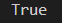My Personal Notes arrow_drop_up
Recommended Articles
Page :